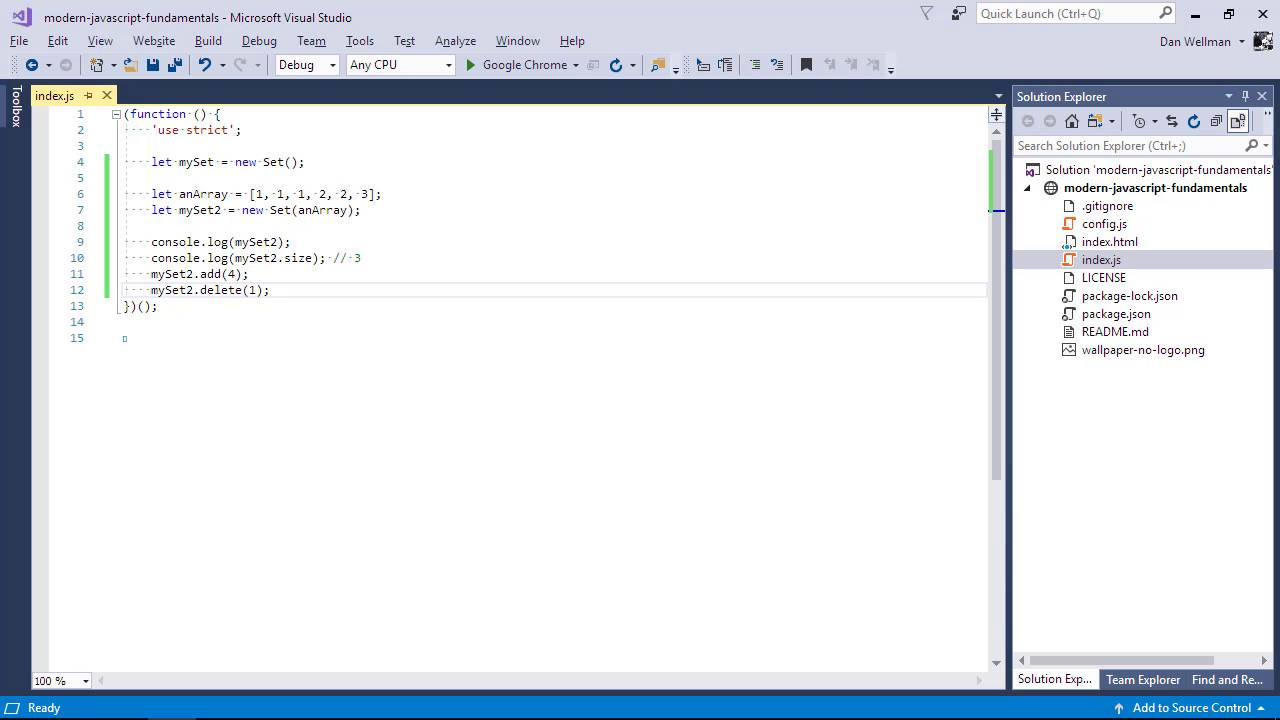Lessons: 65Length: 7.1 hours• Overview
• Transcript

# 3.3 Sets

Sets are JavaScript objects that store unique values of any type. Set is one of the collection classes recently added to JavaScript.

Syntax

• new Set()
• size
• add
• delete
• clear
• has

## 11.Conclusion1 lesson, 00:43

### 3.3 Sets

Hi folks, in this lesson we're going to look at a data structure called a set. A set is similar to an array, but in a set you can only store unique values inside it. The values can be of any type, but they must all be unique. To create a set, we use the set constructor. At this point in time, there is no literal form of sets. We can optionally provide an interable object as an argument to the constructor, and all of the values will be added to the set. An array is an interable object. And we'll look at iterators and iterable objects later in the course. For now, just understand that an array is also an iterable object. So in this case, we declare an array called an Array. And we give it some non-unique values, in this case they're all numbers. And then we create a set called mySet2, and we pass the array to the new set. So, using a set is a great way to de-duplicate an array. So let's just log my set to the console. And let's take a look at that in a browser. And we can see that the set just contains the unique values 1, 2, and 3. It doesn't contain all of the values from the array, because not all of the values were unique. To see how many values there were inside a set, we can use the size property of the set. Now in this case we should see the value 3 and indeed we do. We also have a range of different methods that we can use to work with sets. We can add or remove values from the sets using the add and delete methods respectively. And let's just log the set once again so we can see what changes have happened to it. And we can see that in this case, it now contains the values 2, 3 and 4, instead of 1, 2 ,and 3. Because the values are unique, with the delete method, we pass the value that we want to remove. Not the index of the value that we want to remove. We can only add a single value at a time. But the method returns the set itself. So we can chain it, like this. When we use the delete method this method will return true if the value was deleted, or false if we try to delete a value that doesn't exist. So, if we were to change this expression to this. It would simply return force. It wouldn't throw an error, wven though the value 6 is not contained within the set. If we wanted to determine whether a particular value exists in a set. We can use the has method In this case we should see the value true because my set 2 does have a value of 3 inside it. There are a couple of different ways that we can iterate through the values inside a set. We can use the entries or values methods, which both return an iterator. We'll look at iterators in much more detail later on the course. While a set may seem conceptually similar to an array, we should remember that it isn't. They are completely different data types. The values inside a set don't have an index or a key, so we can't pull out individual values using bracket notation, like we can with arrays. So in this lesson we looked at the new set object, which was introduced in ES 2015. So fairly recently we saw that it is a data structure that allows us to store unique values. We saw that we can get the number of values in the set using the size property, and we look to some of that methods that we can use to work with the sets, including add, delete and has. We also learned that sets are similar to arrays, but they are fundamentally different. In the next lesson, we'll be looking at the map objects. Thanks for watching.

Back to the top